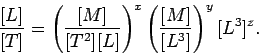Click to Chat

1800-1023-196

+91-120-4616500

CART 0

• 0

MY CART (5)

Use Coupon: CART20 and get 20% off on all online Study Material

ITEM
DETAILS
MRP
DISCOUNT
FINAL PRICE
Total Price: Rs.

There are no items in this cart.
Continue Shopping• Complete JEE Main/Advanced Course and Test Series
• OFFERED PRICE: Rs. 15,900
• View Details

Solved Examples on Unit and Dimensions

Problem 1:-

The speed of sound v in a gas might plausibly depend on the pressure p, the density ρ, and the volume V of the gas. Use dimensional analysis to determine the exponents x, y, and z in the formula

v = CpxρyVz,

where C is a dimensionless constant. Incidentally, the mks units of pressure are kilograms per meter per second squared.

Solution:-

Equating the dimensions of both sides of the above equation, we obtainA comparison of the exponents of [L], [M], and [T] on either side of the above expression yields,

1 = -x – 3y +3z,

0 = x+y,

-1 = -2x.

The third equation immediately gives x = ½ ; the second equation then yields y = – ½ ; finally, the first equation gives z = 0. Hence,Problem 2:-

Milk is flowing through a full pipe whose diameter is known to be 1.8 cm. The only measure available is a tank calibrated in cubic feet, and it is found that it takes 1 h to fill 12.4 ft3.

What is the velocity of flow of the liquid in the pipe'?

Solution:-

velocity is [L]/[t] and the units in the SI system for velocity are therefore m s-1:

v = L/t where v is the velocity.

Now    V = AL where V is the volume of a length of pipe L of cross-sectional area A

i.e. L = V/A.
Therefore v = V/At

Checking this dimensionally

[L][t]-1 = [L]3[L]-2[t]-1 = [L][t]-1

which is correct.

Since the required velocity is in m s-1, volume must be in m3, time in s and area in m2.

From the volume measurement

V/t = 12.4ft3 h-1

We know that,

1 ft3 = 0.0283 m3

1 = (0.0283 m3 /1 ft3)

1 h = 60 x 60 s

So, (1 h/3600 s) = 1

Therefore V/t = 12.4 ft3/h x (0.0283 m3/1 ft3) x (1 h/3600 s)

= 9.75 x 10-5 m3 s-1.

Also the area of the pipe A = πD2/4

= π(0.018)2 /4 m2

= 2.54 x 10-4 m2

v = V/t x 1/A

= 9.75 x 10-5/2.54 x 10-4

= 0.38 m s-1.

Problem 3:-

Force of viscosity F acting on a spherical body moving through a fluid depends upon its velocity (v), radius (r) and co-efficient of viscosity ‘η’ of the fluid. Using method of dimensions obtain an expression for ‘F’.

Solution:-

Let F ∝ va, F ∝ rb and F ∝ ηc                          …... (1)

So, F = Kvarbηc

where ‘K’ is a dimensional constant.

Dimensional formula of  F = [M1L1T-2]

Dimensional formula of   v = [L1T-1]

Dimensional formula of   r = [L1]

Dimensional formula of   η = [M1L-1T-1]

Substitute for the dimensional formulae in equation (1),

[M1L1T-2] = [L1T-1]a [L1]b [M1L-1T-1]c

[M1L1T-2] = [Mc La+b+c T-a-c]                           …... (2)

In accordance to principle of homogeneity, the dimensions of the two sides of relation (2) should be same.

So, c = 1             …... (3)

a+b-c = 1             …... (4)

-a – c = -2            …... (5)

Putting  c = 1 in (5), we get a = 1

Putting a = 1 and c = 1 in (4), we get b = 1

Substituting for a, b and c in (1), F = kηrv, which is required relation.### Course Features

• 728 Video Lectures
• Revision Notes
• Previous Year Papers
• Mind Map
• Study Planner
• NCERT Solutions
• Discussion Forum
• Test paper with Video Solution